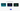# How does the Number and Percentage Outcome work?

The number and percentage outcome type is what most people are familiar with as the result of a quiz. Answers in the quiz are either right or wrong. At the completion of the quiz, the user will be told how many correct answers they achieved, and what percentage this equates to.

For example, if a user answers 15 out of 20 answers correctly, the results page will read "Well done! you scored 15/20. That's 75%!".

For each question you must map as least one answers as correct.